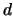Next: The State of the Up: Other Partitioning Algorithms Previous: Reducing the bandwidth of

### Index based algorithms

In a way many of the partitioning algorithms mentioned so far work by generating an ordered list of the vertices and bisect this list into equal segments. In the case of spectral bisection for example, this ordering is induced by the value of the Fiedler vector. There are many classic ways of mapping adimensional regular grid to a one-dimensional list, such that proximate vertices in the original grid are mapped close to each other on the one-dimensional list. These techniques include the Hilbert space-filling curve  and the bit-interleaving transformation . An irregular grid can be so indexed by embedding the grid into a-dimensional regular grid. The advantage of such index based methods is that the ordering can be calculated very quickly. Index based algorithms can also be parallelized and efficiently adopted for situations where vertices are added or deleted as the result of mesh adaptation. The quality of the partitioning is comparable to that of the RCB algorithm .

2000-03-21